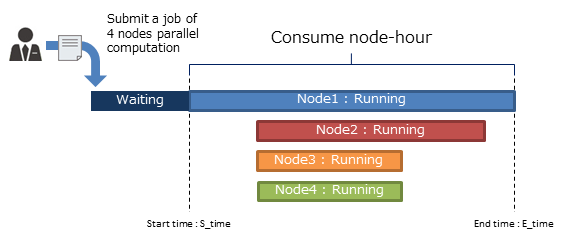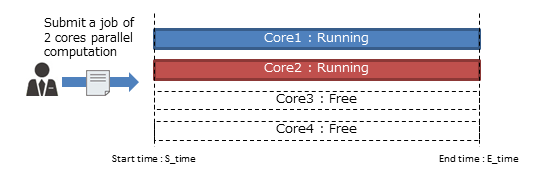This page explains a "node hour" as a unit of resource assignment under shared use and rules.

Under shared use, the amount of computational resources is on the basis of "node-hour"

1 node-hour = the computational resource amount of 1 hour computation using 1 node.
If you have 30 node-hours, you can have one hour computation using 30 nodes or a three-hour computation using 10 nodes.
A node-hour used for computation is reduced from the computational resources assigned to you.

A node-hour is calculated as follows.

Node-hour = number of nodes used for computation × computing time(unit:hour)

"computing time" means the time duration between the computation start and its end.
Computing time is not measured on each node.

For example, in the case of performing a parallel job using 4 nodes,
all nodes use the same computing time as node 1.
Accordingly, a node-hour in this example is calculated as 4 × (E_time - S_time).
This idea is based on the fact that nodes 2, 3, and 4 cannot be used by other users while node 1 is occupied.Also, we calculate a node-hour based on number of nodes. We do not look at the usage ratio of processors in a node.
In short, even if you use only a single core on a multi-core node, this is regarded as using one node.

For example, in the case that 2 cores are used for computation on a node with 4 cores,
you are required to use one node.
Accordingly, the node-hour consumed in this example is 1 × (E_time – S_time).
This idea is based on the fact that our scheduler system deployed on the CMC assigns computational resources to a job request on the basis of a node.You can check your node-hour by using a usage_view command. The output of the command includes the node-hour consumed by your group, and the node-hour consumed by each user of your group. See below for further details:
usage_view command

## Usage Rule for node-hour

・Node-hour is assigned to each group. Node-hour cannot be shared by individual users.

・After consuming all node-hours, it is impossible to submit a job request. As to a computation that is already running, it will finish without being suspended.

・In the case that a job execution is suspended due to system trouble, the node-hour will not be consumed. If this case occurs, we will contact you.